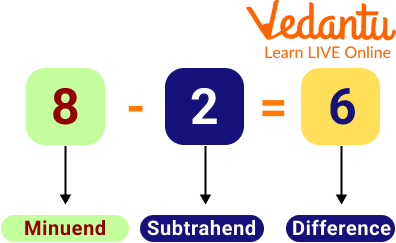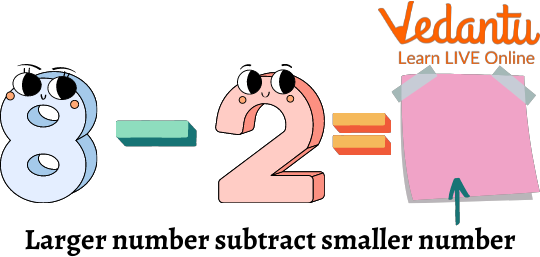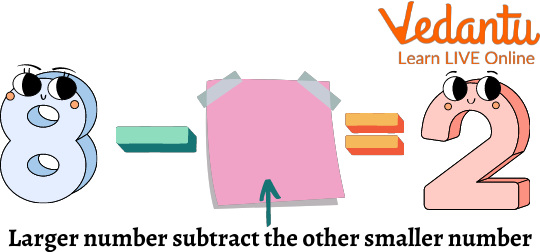Courses
Courses for Kids
Free study material
Offline Centres
More

# 3-Digit Subtraction Missing NumbersLast updated date: 04th Dec 2023
Total views: 102.6k
Views today: 1.02k## Introduction to the Subtraction

Finding the missing numbers in a sequence or a series is a fun activity for the students to identify the patterns and memorise multiplication tables, numbers, fundamental mathematical operations, or even counting. Since subtraction is done by taking away or removing things. It is a way to reduce numbers, operands, or objects. In this article, we will learn missing number subtraction and see how we can do the subtraction when some digits are missing.

## What is Subtraction?

We use subtraction in our everyday life in different situations, like if we buy a toy and it costs 70 rupees, and we give the shopkeeper a 100 rupee note, then to know how much money the shopkeeper will return us, we need to learn the subtraction.

So we can say that it's the operation of removing the objects from the collection.

The subtraction formula is stated as Minuend - Subtrahend = Difference. Here, minuend is the number from which the other number is subtracted, and subtrahend is the number that is subtracted from the minuend. The symbol (-) indicates the subtraction operation, and the difference indicates the result. The example given below shows the subtraction formula.Subtraction parts

## What is Missing Number Subtraction?

Missing numbers in subtraction are those that have been missed in a particular subtraction. We can find those missing numbers by identifying the pattern. Whenever there are blanks in a series of numbers, a few numbers have been missed, and we need to observe the pattern and fill in the blanks with suitable numbers. We can find missing numbers to memorise counting and multiplication tables and learn arithmetic operations.

## How to Solve Missing Digit Subtraction?

It's very interesting to learn about missing number subtraction.

In missing number subtraction probably, we will be provided with 3 cases which will be very easy to solve, and they will be solved in the same way whether its column based or given in single digits. So now let's learn about each case one by one in detail.

Case 1: In the first case, we can see that if the missing number is immediately after the equals sign, we need to subtract the second number from the first.Missing Digit Subtraction

We need to find a number that is equal to 8 minus 2. When the number 2 is subtracted from 8.

So, we have 8 - 2 = 6.

Case 2: In the second case, we can see that if the missing number is being subtracted from the larger number, we need to subtract the given answer from the larger number.Missing Digit Subtraction

We need to find a number which when subtracted from 8, gives the difference equal to 2. So, 8 - 6 = 2

Case 3: If the missing number is immediately before the subtraction sign, we need to add the two numbers together.Missing digit subtraction

Therefore, as per the above image, we have

_____ - 6 = 2

So, on adding 6 + 2, we get 8. And if 6 is subtracted from 8, we get 2.

Hence, the missing number is 8 here.

## Important Points to Keep in Mind While Doing Subtraction

• If Zero is subtracted from a number, it does not change the value of the number.

• If 1 is subtracted from a number, then it gives the predecessor of the number as the difference.

• The number from which the other number is to be subtracted is called the minuend, The number subtracted is called the subtrahend, and the answer we get is called the difference.

• If the subtrahend is missing, then the difference is subtracted from the minuend.

• If we want to find the missing minuend, the difference is added to the subtrahend.

• Difference + subtrahend = minuend

## Solved Examples

(i) 9 _ = 4

Ans. The number, which is taken away from 9, so that it leaves 4 as the difference, is 5.

Therefore, the missing digit should be written as 9 – 5 = 4

(ii)? 2 = 5

Ans. Finding the number that must be subtracted by 2 to leave the number 5.

Therefore, the missing number should be 7 – 2 = 5.

(iii) 6 - _ = 5

Ans. Finding out the number which is taken away from 6 so that it leaves 5

Therefore, the solution should be written as 6 – 1 = 5.

## Practice Questions

Q 1. __ - 6 = 6 (Ans: 12)

Q 2. 8 - __= 2 (Ans: 6)

Q 3. 17 - 8 =__ (Ans: 9)

Q 4. 23 - 5 =__ (Ans: 18)

Q 5. __ - 13 = 3 (Ans: 16)

## Summary

So in this article, we learned about so many interesting things which were related to subtraction, like what subtraction and missing number subtraction is, how to use them in our everyday life, what are the various forms of missing digit subtraction, how to solve them and also learned that what are the important points to keep in mind while doing subtraction. We read about it and solved some examples to clear all your doubts about the concepts.

## FAQs on 3-Digit Subtraction Missing Numbers

1. What are 3 keywords that are used for subtraction?

Minus, take away, and fewer than are the 3 keywords for subtraction.

2. What are the advantages of missing numbers activities?

The activity of finding missing numbers helps the students to develop mental skills and adds to their fine motor practice of learning.

3. Who invented subtraction?

Brahmagupta of India invented the arithmetic operation subtraction.